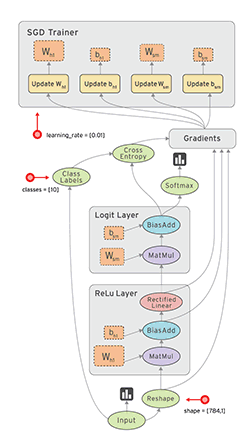DAY 3
0
Google Developers Machine Learning

[Day03] How Google does Machine Learning介紹

機器學習在GCP上運作的流程2.學習用Tensorflow來試著建立你的機器學習模型
3.進行機器學習的特徵工程
4.以分佈式的方式端到端建立機器學習模型
5.機器學習的應用技巧，運用在處理圖像或文本、序列模型或是分類模型，甚至是建立個性化推薦系統等

Tensorflow

Tensorflow使用開源的方式讓大家都能使用，加速整個深度學習的發展，Tensorflow可以使用在CPU、GPU、TPU上面，Tensorflow是利用資料流程圖來表達數值運算的開放式原始碼函式庫，可以拆分成Tensor與Flow兩種來解釋：

• Tensor：張量，是一個n維度的陣列或列表，一維Tensor是向量，二維Tensor是矩陣
• Flow：指Graph運算過程中的資料流hello world

import tensorflow as tf
hello = tf.constant('Hello World')
sess = tf.Session()
sess.run(hello)

兩個矩陣相乘

import tensorflow as tf
c = tf.constant([[1.0, 2.0], [3.0, 4.0]])
d = tf.constant([[1.0, 1.0], [0.0, 1.0]])
e = tf.matmul(c, d)
with tf.Session() as sess:
result = sess.run(e)
print(result)

Keras

Keras也是和Tensorflow在同一年出現，它可以接合Tensorflow或Theano等等深度學習框架後端，簡單直觀易上手的特性深受大眾喜愛，使用者很容易用幾行程式就能建立神經網路，相比Tensorflow來得簡單許多。

from keras.models import Sequential
from keras.layers import Dense, Activation
model = Sequential()
model.compile(optimizer='rmsprop',
loss='mse')

Pytorch

class Net(nn.Module):
def __init__(self):
super(Net, self).__init__()
self.conv1 = nn.Conv2d(3, 32, 3)
self.conv2 = nn.Conv2d(32, 16, 3)
self.fc1 = nn.Linear(16 * 6 * 6, 10)
self.pool = nn.MaxPool2d(2, 2)
def forward(self, x):
x = self.pool(F.relu(self.conv1(x)))
x = self.pool(F.relu(self.conv2(x)))
x = x.view(-1, 16 * 6 * 6)
x = F.log_softmax(self.fc1(x), dim=-1)
return x
model = Net()